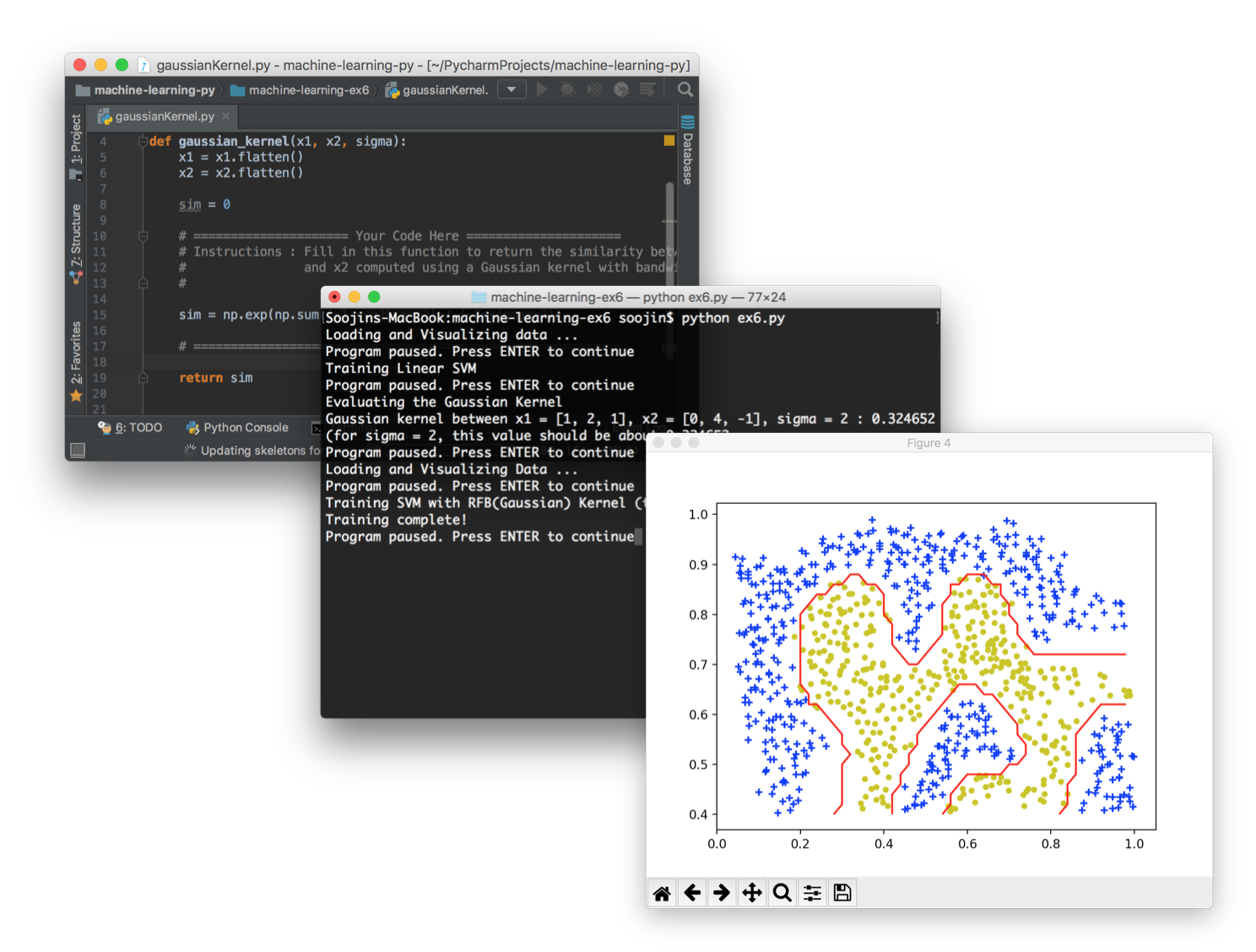# Coursera Machine Learning Assignments in PythonIf you've finished the amazing introductory Machine Learning on Coursera by Prof. Andrew Ng, you probably got familiar with Octave/Matlab programming. With this repo, you can re-implement them in Python, step-by-step, visually checking your work along the way, just as the course assignments.

## How to start

### Dependencies

This project was coded in Python 3.6

• numpy
• matplotlib
• scipy
• scikit-learn
• scikit-image
• nltk

### Installation

The fastest and easiest way to install all these dependencies at once is to use Anaconda.

## Important Note

There are a couple of things to keep in mind before starting.

• all column vectors from octave/matlab are flattened into a simple 1-dimensional ndarray. (e.g., y's and thetas are no longer m x 1 matrix, just a 1-d ndarray with m elements.) So in Octave/Matlab,
``````>> size(theta)
>> (2, 1)
``````
Now, it is
``````>>> theta.shape
>>> (2, )
``````
• numpy.matrix is never used, just plain ol' numpy.ndarray

## Contents

#### Exercise 1

• Linear Regression
• Linear Regression with multiple variables

#### Exercise 2

• Logistic Regression
• Logistic Regression with Regularization

#### Exercise 3

• Multiclass Classification
• Neural Networks Prediction fuction

#### Exercise 4

• Neural Networks Learning

#### Exercise 5

• Regularized Linear Regression
• Bias vs. Variance

#### Exercise 6

• Support Vector Machines
• Spam email Classifier

#### Exercise 7

• K-means Clustering
• Principal Component Analysis

#### Exercise 8

• Anomaly Detection
• Recommender Systems

## Solutions

You can check out my implementation of the assignments here. I tried to vectorize all the solutions.

Get A Weekly Email With Trending Projects For These Topics
No Spam. Unsubscribe easily at any time.
Python (807,639
Matlab (31,164
Neural Network (15,509
Coursera (2,492
Octave (1,754
Logistic Regression (1,560
Anomaly Detection (1,028
Ndarray (575
Support Vector Machines (509
Principal Component Analysis (195
Coursera Machine Learning (45
Andrew Ng Course (42
Andrew Ng Machine Learning (19
Numpy Exercises (12
Andrew Ng Ml Course (6
Python Ml (4